American Journal of Theoretical and Applied Statistics
Volume 5, Issue 1, January 2016, Pages: 23-26

Commentary

A Review on ‘Probability and Stochastic Processes’

Bodo Herzog1, 2, 3

1Department of Economics, Reutlingen University, Reutlingen, Germany

2ESB Business School, Reutlingen University, Reutlingen, Germany

3RRI Reutlingen Research Institute, Reutlingen, Germany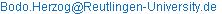Bodo Herzog. A Review on ‘Probability and Stochastic Processes’. American Journal of Theoretical and Applied Statistics. Vol. 5, No. 1, 2016, pp. 23-26. doi: 10.11648/j.ajtas.20160501.14

Abstract: This paper is a commentary on the book ‘Probability and Stochastic Processes’ from Ionut Florescu. The book is an excellent introduction to both probability theory and stochastic processes. It provides a comprehensive discussion of the main statistical concepts including the theorems and proofs. The introduction to probability theory is easy accessible and a perfect starting point for undergraduate students even with majors in other subjects than science, such as business or engineering. The book is also up-to-date because it includes programming code for simulations. However, the book has some weaknesses. It is less convincing in more advanced topics of stochastic theory and it does not include solutions to excises and recent research trends.

Keywords: Probability Theory, Stochastic Processes, Simulation of Stochastic Processes

Contents

1. Introduction

The book ‘Probability and Stochastic Processes’ by Ionut Florescu  is certainly not recognized as one of the big surprises in the recent book market. The book has nearly 500 pages and is a standard textbook of probability theory and stochastic processes [2, 3, 4]. But despite its length and sophisticated mathematical topics, it is an excellent exposition in a highly interesting field that becomes more and more relevant in almost all modern scientific disciplines. It is a relevant subject in engineering but similarly in social sciences such as economics or sociology. But recent research also recognizes that these topics are highly important in natural sciences such as physics, biology or psychology because natural laws are rather probabilistic than deterministic.

Of course, one reason why the book is not recognized by a broad audience is the mathematical subject. Nevertheless, the book is written in an easy language and it is even accessible for undergraduate students without prior advanced mathematical skills. The huge range of topics provides a good introduction and overview to all elementary topics in probability theory.

In the next two sections, I describe the structure of the book and discuss the most important differences to other textbooks in that field. Section 4 concludes the paper.

2. Structure of the Book

The main theme of the book is the introduction to basic statistics, probability theory and stochastic processes. However, the author also touches on more advanced topics such as stochastic differential equations at the end.

If you start reading a book on probability theory, usually you begin with basic definitions of a probability space, probability measure and set theory. At first, the outline of the book by Ionut Florescu seems to be no exception here. But surprisingly, the introduction section, before any mathematical discussion starts, is about a brief history of probability theory. Actually this makes the book certainly different to other books in the field. Hence, it is much more readable for undergraduate and graduate students from other scientific fields. The brief description of the historical evolution of probability theory over centuries is really motivating to readers at the beginning. The reader gets a good overview over the notions in the subject and the leading scholars in this field of study. There is no doubt this first positive impression is due to the well-thought book structure.

Part I of the book provides the introduction to probability theory. It is divided in 8 chapters that contain the elementary tools from definitions of probability measures, random variables up to integration theory, conditional expectation, probability distribution, characteristic function and limit theorems. It is needless to say that the author does not discuss all definitions from a philosophical vantage point. Otherwise, he has to say that definitions are neither right nor wrong – they just have to be applicable. Thus, any definition has advantages and disadvantages. As long as this discussion is currently not typical in a statistic textbook for beginners, it is not problematic for students without prior knowledge.

Part II of the book discusses more advanced issues such as stochastic processes. It covers the definitions such as the idea of a filtration, martingale, Brownian motion, Poisson process or Markov process. Towards the end, the author touches on more advanced and important topics, such as the solution of stochastic differential equations (SDEs), the application and simulation of SDEs in economics as well as the linkage to partial differential equations (PDEs).

Next let me explain what is really different in this book and why is it a recommendable reading? And even more importantly, what can we learn from it? In the following you can read a brief book commentary in a fully objective manner and to my advantage: I do not know the author.

3. Review of the Book

3.1. Positive Aspects of the Book

First of all, Florescu’s book is based on a scientific approach and uses an almost standard structure to introduce probability theory and stochastic processes. Of course there are already seminal books on both topics in the book market [2, 3, 4]. But the book under review differentiates to standard books at some point and this unique difference makes the book well readable for students of all scientific fields. One of these differences is already mentioned and concerns the brief historical exposition to probability theory in the first section of the book. This is an excellent overview and motivating for readers at the same time in particular for a book with almost 500 pages of rather mathematical theory.

Moreover, Florescu introduces the definitions in a sensible way with comprehensive explanations, proofs and importantly for students including numerous examples and take-home problems. He is quite frank about limitations and discusses several practical problems and paradoxes, such as the famous Monty Hall problem in elementary statistics.

In the Monty Hall problem, there is a host of a TV show that first gives you the choice between three closed doors to win some price. Two of them have nothing behind, and one contains a prize. After choosing one door, the door is at first not opened immediately. Instead the host opens another door that contains nothing. Now the host gives you the chance of changing your choice or sticking with your initial door. Most people would stick with the initial choice because they believe it does not matter. However, that is not true. This nicely demonstrates the importance of probability theory in practice. The reader can easily understand why this is the case. Actually, it turns out that it is always better to switch the door.

There are many other interesting examples in the book, such as De Mére’s paradox, Bertrand’s paradox, the Gambler’s ruin problem, the birthday problem and so on. The balance of pure theory and discussion as well as examples is impressive and helps the reader to get a better understanding of all the insights of probability theory.

A second secret of Florescu’s book is that he avoids at the beginning and even later in the book some sophisticated mathematical details. In my opinion, however, it is also a weakness because it displays some concepts as finished and makes it difficult for the reader to imagine why advanced concepts and research take place up until today.

The third secret of the book is the use of graphical illustrations and even programming code to replicate the illustrations in case of more sophisticated problems. Here the reader really benefits in contrast to other books. This confirms that the author has the idea that a book on probability theory must be easy accessible to everyone and it must be designed based on learning by doing. Last but not least, if there are deeper technical discussions they are represented in a structured manner.

In a nutshell, the book is nicely balanced. It is published for students and professionals in all scientific fields. In addition, it is a good introduction to probability theory in a do-it-yourself fashion. Overall, Florescu wrote an excellent book but it is not a book for research oriented scholars. They find only limited reference to scientific papers or current research issues such as in the seminal book by  on Brownian Motion and Stochastic Calculus. Moreover, he mainly introduces standard theories and does not take into account new developments in probability theory or related fields, such as Lie algebra . All in all, the positive impression outweighs.

3.2. Negative Aspects of the Book

Let me list some issues that are not discussed as comprehensive as expected in this book.

The second part of the book is on stochastic processes. In this part the book is not as deep as in the first part in particular on continuous time processes, such as continuous martingale theory. However, continuous martingale theory is an important concept in theory and application in finance and for stochastic integration and partial differential equations. These are topics the book touches at the end.

The study of Brownian motion is rather brief and does not contain the deep and important aspects that it plays in practical applications for the solution of stochastic or partial differential equations until today . Despite the R code is provided by the author, interesting stochastic simulations are either not included or omitted probably due to page restrictions. Of course, students or readers without R knowledge look for more support at that point and maybe a starter kid for other programming languages especially research related once, such as MATLAB . Of course, R has a big advantage. The R program is open source while MATLAB is not. In fact, the code can easily be translated into MATLAB, however, the potential target group of the book probably does not have the sufficient skills in doing so without hints. Let me briefly illustrate and discuss three topics missing in the book, despite all of this is rather basic and introductory.

First of all, let me demonstrate the issue of scaling of a random process, such as a random walk discussed in chapters 3 and 12. Scaling is actually an important topic in science in particular in finance because high frequency data has other properties than annual data. And that is not due to differences in the fundamentals, it is mainly due to scaling. Figure 1 demonstrates this issue by simulation.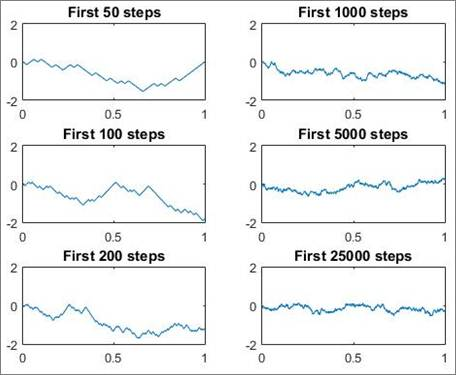Figure 1. Scaling of a Random Process. Source: own simulation.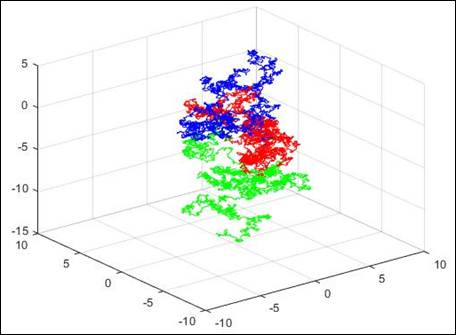Figure 2. 3D-Brownian Motion, simulation for 50 steps and 3 particles. Source: own simulation.

On the upper left panel you see a random process with 50 steps and on the bottom right panel the same process with 25000 steps. Hence, it is already important for beginners to see and learn the impact of scaling. Usually high-frequency data look like the bottom right panel and thus contain less variance than in the upper left panel although both data are random processes.

The second issue is a brief study of multi-dimensional Brownian motions. The book mainly focuses on one-dimensional Brownian motions in particular in case of simulation. However, even without any rigorous mathematical discussion of a 3-dimensional Brownian motion, it is not too difficult to do some simulations (Figure 2). The simulation of a 3-dimensional Brownian motion helps the reader to get a better and more general impression of stochastic processes. Figure 2 displays a 3-dimensional Brownian motion of 3 moving particles over 50 steps.

Finally, let me discuss the simulation of the backward and forward Kolmogorov equation [9, 10]. This equation is important in almost all applied sciences and also referred to Fokker-Planck equation. Suppose you look for the solution of parabolic partial differential equations of the form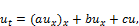(1)

whereand boundary values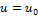at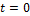and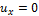for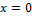and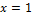. The respective solution or simulation of the problem provides important insides. In fact, in theory we distinguish between the backward Kolmogorov equation (top panel, Figure 3) and the forward Kolmogorov equation (bottom panel, Figure 3). The Figures demonstrate the spatial flow over time and the respective probability density functions.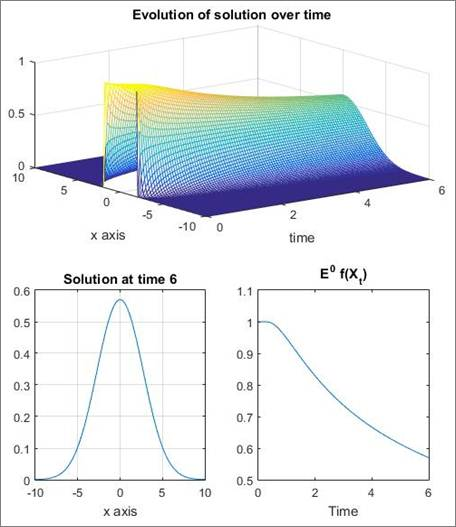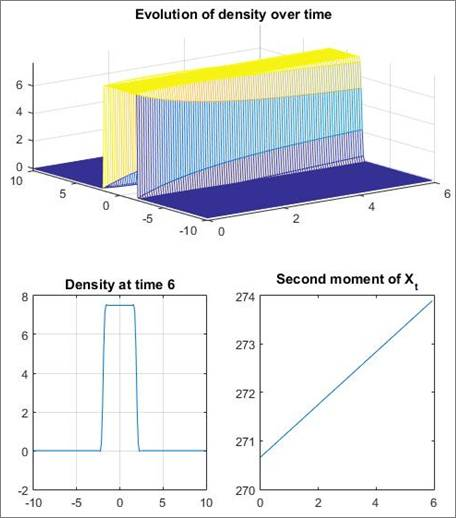Figure 3. Backward Kolmogorov (top) and Forward Kolmogorov (bottom). Source: own simulation.

Part II of the book contains definitely some weaknesses in respect of the stringency and deepness of stochastic analysis. The uninformed reader may get the impression that stochastic theory is somewhat ‘general store’ like. Of course, the advanced discussion is probably excluded due to the fact that the book has already almost 500 pages. From a beginner’s perspective, however, all these weaknesses are less important.

However, a beginner would complain about a little other point. In a world of open access it is not reasonable why the reader does not get full and free access to all the solutions of problems in the book. I strongly believe the book will gain if the author includes the solutions either in the appendix or prepares an solution book for the numerous of problems.

4. Conclusion

In summary, Part I and Part II of the book are a stimulating introduction to the theory of probability and stochastic processes. However, the second part of the book is less deep in terms of theory and its implications especially in respect of continuous stochastic processes, the solution and simulation of stochastic differential equations and its linkage to partial differential equations. Nevertheless, the overall impression of the book is positive. Therefore, I recommend this book for students who want to get an introduction to probability theory. In addition, the book can be used as a handbook. Professionals may use it to recap or relearn probability concepts that are applied on a daily basis in practice. As a result, Florescu's work is a good reference and introduction to probability theory and stochastic processes.

Acknowledgements

I’m grateful for financial support by the Reutlingen Research Institute (RRI). Finally, I’m grateful to the comments from two anonymous referees. All remaining errors are my responsibility.

References

1. Florescu, I., Probability and Stochastic processes, John Wiley & Sons, 1 ed., 2015.
2. Yates, R.D. and Goodman, D.J., Probability and Stochastic Processes, John Wiley & Sons, 2004.
3. Yates, R.D. and Goodman, D.J., Probability and Stochastic Processes: A Friendly Introduction for Electrical and Computer Engineers, John Wiley & Sons, 2014.
4. Parzen, E., Stochastic Processes, Dover Publications, 2015.
5. Karatzas, I. and Shreve, S.E., Brownian Motion and Stochastic Calculus, Springer Press, 1998.
6. Baldeaux, J. and Platen, E., Functionals of Multidimensional Diffusions with Applications to Finance, Spring Press, 2013.
7. Yang, X., On the large deviation principle of generalized Brownian bridges, Journal of Mathematical Analysis and Applications, Vol. 430, No. 2, p. 845-856, 2015.
8. Guenther, M. and Juengel, A., Finanzderivate mit MATLAB, Mathematische Modellierung und numerische Simulation, Vieweg-Teubner Verlag, 2010.
9. Okstendal, B., Stochastic Differential Equations, Spring Press, 2003.
10. Holden et al., Stochastic Partial Differential Equations, Springer Press, 2010.

 Contents 1. 2. 3. 3.1. 3.2. 4.
Article ToolsAbstractPDF(652K)